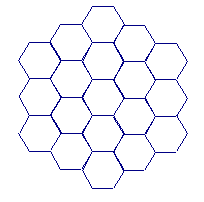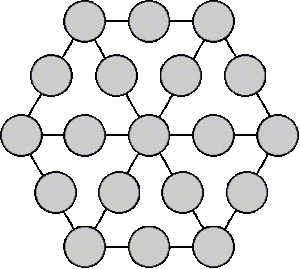# Magic Hexagon

Make the sum of each of the rows equal certain numbers.

In the hexagons below, place the numbers 1-19 so that rows, columns and diagonals all add up to 38.(Source: Eric W. Weisstein)Extension:
Place the numbers 1-19 in the circles so the sum of each of the rows with three circles around the outside is 23 and the sum of each of the rows with five circles through the middle is 40.(Source: Jon Basden)